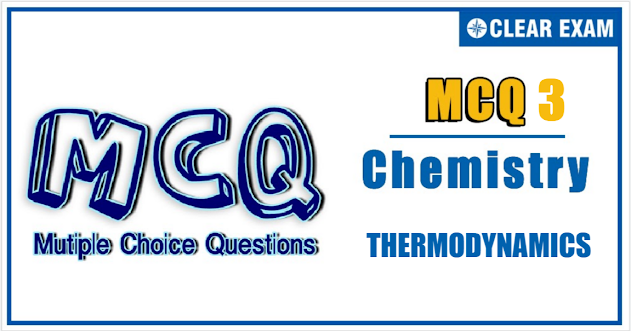## [LATEST]\$type=sticky\$show=home\$rm=0\$va=0\$count=4\$va=0

IIT JEE exam which consists of JEE Main and JEE Advanced is one of the most important entrance exams for engineering aspirants. The exam is held for candidates who are aspiring to pursue a career in the field of engineering and technical studies.
Chemistry is important because everything you do is chemistry! Even your body is made of chemicals. Chemical reactions occur when you breathe, eat, or just sit there reading. All matter is made of chemicals, so the importance of chemistry is that it's the study of everything.
.

Q1.Which is not intensive property?:
•  Boiling point
•  Refractive index
•  Molarity
•  Volume
Solution
Volume
Q2.Which one of the following statements is false?
•  Work is a state function
•  Temperature is a state function
•  Change in the state is completely defined when the initial and final states are specified
•  Work appears at the boundary of the system
Solution
Work is a state function
Q3.The ∆fH for CO2(g), CO(g), and H2O(g)are -393.5, -110.5, and-214.8 kJ mol-1, respectively.
The standard enthalpy change (in kJ mol-1) for the reaction
CO2(g)+H2(g)⟶CO(g)+H2O(g) is
•  +524.1
•  +41.2
•  -262.5
•  -41.2
Solution
CO2(g)+H2(g)⟶CO(g)+H2O(g)
∆H={[∆fHCO, (g)+∆fHH2O, (g)] -∆fHCO2, (g)}
= [-110.5+(-241.8(--393.5+0]
= +41.2 kJ
fHH2, (g) = 0
fH = 0 in elementary state

Q4.If ∆fH of ICl(g), Cl(g), and I(g)) is 17.57, 121.34 and 106.96 J mol-1 respectively. Then bond dissociation energy of I-Cl bond is
•  35.15 J mol-1
•  106.69 J mol-1
•  210.73 J mol-1
•  420.9 J mol-1
Solution
210.73 J mol-1

Q5.The difference between the heats of reaction at constant pressure and constant volume for the reaction
2C6H6(l)+15O2⟶12CO2(g)+6H2O(l) at 25℃ in kJ is
•  -7.43
•  +3.72
•  -3.72
•  +7.43
Solution
2C6H6(l)+15O2⟶12CO2(g)+6H2O(l)
∆H = ∆E + ∆ngRT
∆H - ∆E = ∆ngRT
∆ng = 12 - 15 = -3 ∆H - ∆E = -3×8.314×298 = -7432.716 J

Q6.The heat of hydrogenation of ethane is x1 and that of benzene is x2
Hence resonance energy of benzene is
•  x1 - x2
•  x1 + x2
• 3x1 - x2
•  x1-3x2

Q7.The relationship between the free energy change (∆G) and entropy change (∆S) at constant temperature (T) is
•  ∆G = ∆H - T∆S
•  ∆H = ∆G + T∆S
•  T∆S = ∆G + ∆H
•  ∆G = -∆H - T∆S
Solution
∆G = ∆H - T∆S

Q8.Molar heat capacity of water in equilibrium with ice at constant pressure is
•  Zero
•  Infinity
•  40.45 kJ K-1mol-1
•  75.48 J K-1mol-1
Solution
By definition CP,m=dqp/dT
For H2O(l)⇌H2O(s),
Temperature does not change if some heat is given to the system. Hence
CP,m=+ve/Zero=∞

Q9.H2+ 1/2O2 → H2O; ∆H=-68 kcal
K + H2O + aq → KOH(aq)+1/2H2; ∆H=-48 kcal
KOH + aq → KOH(aq);∆H = -14 kcal
From the above data, the standard heat of formation of KOH in kcal is
•  -68 + 48 - 14
•  -68 -48 + 14
•  68 - 48 + 14
•  68 + 48 + 14
Solution
-68 -48 + 14

Q10. If a gas absorbs 200 J of heat and expands by 500 cm3 against a constant pressure of 2×105 N m-2, then the change in internal energy is
•  -300 J
•  -100 J
•  +100 J
•  +300 J
Solution
∆U = q - w = q - P∆V
=200-2×105×500×10-6
=100 J#### Written by: AUTHORNAME

AUTHORDESCRIPTION## Want to know more

Please fill in the details below:

## Latest NEET Articles\$type=three\$c=3\$author=hide\$comment=hide\$rm=hide\$date=hide\$snippet=hide

Name

ltr
item
BEST NEET COACHING CENTER | BEST IIT JEE COACHING INSTITUTE | BEST NEET & IIT JEE COACHING: THERMODYNAMICS Quiz-3
THERMODYNAMICS Quiz-3
https://1.bp.blogspot.com/-cARFX6HaUdg/YNyr7ZnqniI/AAAAAAAAPbQ/WmXEnzIKh48dqaaPwpBN6pyzuiTpoUluQCLcBGAsYHQ/w631-h331/quiz%2B3.PNG
https://1.bp.blogspot.com/-cARFX6HaUdg/YNyr7ZnqniI/AAAAAAAAPbQ/WmXEnzIKh48dqaaPwpBN6pyzuiTpoUluQCLcBGAsYHQ/s72-w631-c-h331/quiz%2B3.PNG
BEST NEET COACHING CENTER | BEST IIT JEE COACHING INSTITUTE | BEST NEET & IIT JEE COACHING
https://www.cleariitmedical.com/2021/07/thermodynamics-quiz-3.html
https://www.cleariitmedical.com/
https://www.cleariitmedical.com/
https://www.cleariitmedical.com/2021/07/thermodynamics-quiz-3.html
true
7783647550433378923
UTF-8

STAY CONNECTED